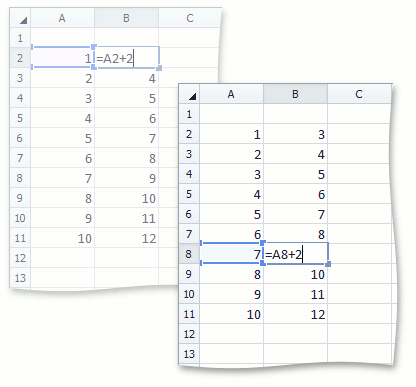# How to: Create Shared Formulas

This example demonstrates how to apply a shared formula in a range of cells to avoid duplicating the same formula for each cell in the range, and reduce the size of the spreadsheet file. To do this, assign the formula string to the CellRange.Formula property of the cell range. The shared formula will be created and applied automatically.

``````worksheet.Cells["A2"].Value = 1;

// Use the shared formula in the "A3:A11" range of cells.
worksheet.Range["A3:A11"].Formula = "=SUM(A2+1)";

// Use the shared formula in the "B2:B11" range of cells.
worksheet.Range["B2:B11"].Formula = "=A2+2";
``````

The following image shows the result of executing the code above.